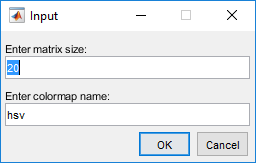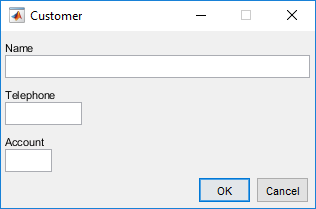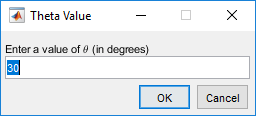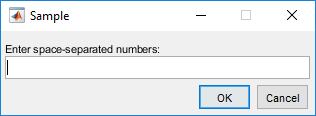# inputdlg

## 语法

answer = inputdlg(prompt)
answer = inputdlg(prompt,dlgtitle)
answer = inputdlg(prompt,dlgtitle,dims)
answer = inputdlg(prompt,dlgtitle,dims,definput)
answer = inputdlg(prompt,dlgtitle,dims,definput,opts)

## 说明

answer = inputdlg(prompt) 创建包含一个或多个文本编辑字段的模态对话框，并返回用户输入的值。返回值是字符向量元胞数组的元素。元胞数组的第一个元素对应于对话框顶部编辑字段中的响应。第二个元素对应于下一个编辑字段中的响应，依此类推。

answer = inputdlg(prompt,dlgtitle) 指定对话框的标题。

当 dims 是标量值时，answer = inputdlg(prompt,dlgtitle,dims) 指定每个编辑字段的高度。当 dims 是数组时，每个数组元素中的第一个值设置编辑字段的高度。每个数组元素中的第二个值设置编辑字段的宽度。

answer = inputdlg(prompt,dlgtitle,dims,definput) 指定每个编辑字段的默认值。definput 输入参数包含的元素数量必须与 prompt 相同。

answer = inputdlg(prompt,dlgtitle,dims,definput,opts) 指定当 opts 设置为 'on' 时，对话框可在水平方向调整大小。当 opts 是结构体时，它指定对话框是否可在水平方向调整大小、是否为模态，以及是否解释 prompt 文本。

## 示例

prompt = {'Enter matrix size:','Enter colormap name:'}; dlgtitle = 'Input'; dims = [1 35]; definput = {'20','hsv'}; answer = inputdlg(prompt,dlgtitle,dims,definput)x = inputdlg({'Name','Telephone','Account'},... 'Customer', [1 50; 1 12; 1 7]);• 包含 \theta 字符序列的提示

• 对话框标题

• 默认输入值为 30

• 编辑字段高度等于 1 个文本行，宽度等于 40 个字符单元

• TeX 作为解释器

prompt = {'Enter a value of \theta (in degrees)'}; dlgtitle = 'Theta Value'; definput = {'30'}; dims = [1 40]; opts.Interpreter = 'tex'; answer = inputdlg(prompt,dlgtitle,dims,definput,opts);answer = inputdlg('Enter space-separated numbers:',... 'Sample', [1 50])user_val = str2num(answer{1}) 
user_val = 1 2 3 4 -5 13

## 输入参数

• 如果 dims 是标量，则它指定所有编辑字段的高度。高度是两个文本行的基线之间的距离。所有编辑字段的宽度是对话框允许的最大宽度。

• 如果 dims 是列向量或行向量，则每个元素指定对话框中从上到下每个对应编辑字段的高度。所有编辑字段的宽度是对话框允许的最大宽度。

• 如果 dims 是数组，则其大小必须为 m×2，其中 m 为对话框中的提示数量。每一行指向对应提示的编辑字段。第一列指定文本行中编辑字段的高度。第二列指定该编辑字段的宽度（以字符为单位）。使用系统字体时，字符单元的宽度等于字母 x 的宽度。

Resize

'off'（默认值）或 'on'。如果设置为 'off'，则用户不能调整对话框大小。如果设置为 'on'，则用户可以水平调整窗口大小。

WindowStyle

'modal'（默认值）或 'normal'。如果设置为 'modal'，则用户必须先做出响应，然后才能与其他窗口交互。

Interpreter

'none'（默认值）或 'tex'。如果设置为 'tex'，则使用 TeX 呈现提示。对话框标题不受影响。

^{ }上标'text^{superscript}'
_{ }下标'text_{subscript}'
\bf粗体'\bf text'
\it斜体'\it text'
\sl伪斜体（通常与斜体相同）'\sl text'
\rm常规字体'\rm text'
\fontname{specifier}字体名称 - 将 specifier 替换为字体系列的名称。您可以将此说明符与其他修饰符结合使用。'\fontname{Courier} text'
\fontsize{specifier}字体大小 - 将 specifier 替换为以磅为单位的数值标量值。'\fontsize{15} text'
\color{specifier}字体颜色 - 将 specifier 替换为以下颜色之一：redgreenyellowmagentablueblackwhitegraydarkGreenorangelightBlue'\color{magenta} text'
\color[rgb]{specifier}自定义字体颜色 - 将 specifier 替换为三元素 RGB 三元组。'\color[rgb]{0,0.5,0.5} text'

\alpha

α

\upsilon

υ

\sim

~

\angle

\phi\leq

\ast

*

\chi

χ

\infty

\beta

β

\psi

ψ

\clubsuit

\gamma

γ

\omega

ω

\diamondsuit

\delta

δ

\Gamma

Γ

\heartsuit

\epsilon

ϵ

\Delta

Δ

\spadesuit

\zeta

ζ

\Theta

Θ

\leftrightarrow

\eta

η

\Lambda

Λ

\leftarrow

\theta

θ

\Xi

Ξ

\Leftarrow

\vartheta

ϑ

\Pi

Π

\uparrow

\iota

ι

\Sigma

Σ

\rightarrow

\kappa

κ

\Upsilon

ϒ

\Rightarrow

\lambda

λ

\Phi

Φ

\downarrow

\mu

µ

\Psi

Ψ

\circ

º

\nu

ν

\Omega

Ω

\pm

±

\xi

ξ

\forall

\geq

\pi

π

\exists

\propto

\rho

ρ

\ni

\partial

\sigma

σ

\cong

\bullet

\varsigma

ς

\approx

\div

÷

\tau

τ

\Re

\neq

\equiv

\oplus

\aleph

\Im

\cup

\wp

\otimes

\subseteq

\oslash

\cap

\in

\supseteq

\supset

\lceil

\subset

\int

\cdot

·

\o

ο

\rfloor

\neg

¬

\nabla

\lfloor

\times

x

\ldots

...

\perp

\surd

\prime

´

\wedge

\varpi

ϖ

\0

\rceil

\rangle

\mid

|

\vee

\langle

\copyright

## 提示

• 即使模态输入对话框处于活动状态，MATLAB 程序也会继续执行。要阻止程序执行直到用户做出响应，可以使用 uiwait 函数。

input = str2num(answer{1}) input = 1.0000 2.0000 3.0000 4.0000 -5.0000 6.0000 + 7.0000i